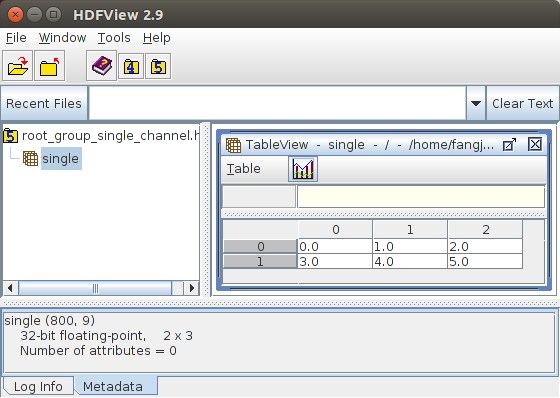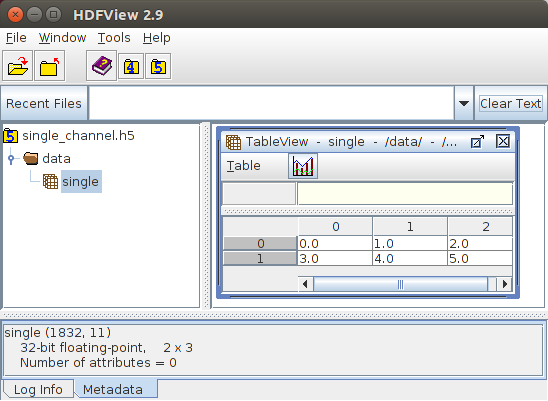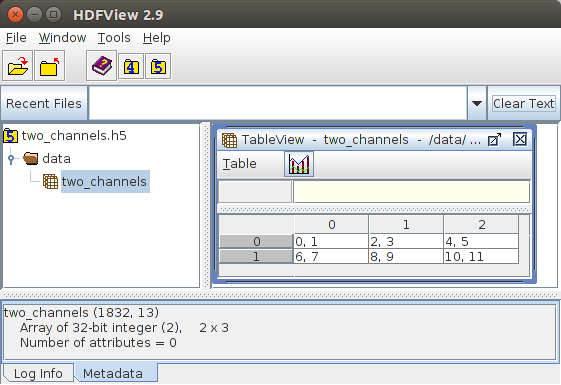OpenCV  4.0.0-beta Open Source Computer Vision

## Goal

This tutorial shows you:

• How to create a dataset?
• How to write a cv::Mat to a dataset?
• How to read a cv::Mat from a dataset?
Note
Currently, it supports only reading and writing cv::Mat and the matrix should be continuous in memory. Supports for other data types have not been implemented yet.

## Source Code

The following code demonstrates writing a single channel matrix and a two-channel matrix to datasets and then reading them back.

You can download the code from here or find it in the file modules/hdf/samples/create_read_write_datasets.cpp of the opencv_contrib source code library.

#include <iostream>
#include <opencv2/core.hpp>
#include <opencv2/hdf.hpp>
using namespace cv;
static void write_root_group_single_channel()
{
String filename = "root_group_single_channel.h5";
String dataset_name = "/single"; // Note that it is a child of the root group /
// prepare data
Mat data;
data = (cv::Mat_<float>(2, 3) << 0, 1, 2, 3, 4, 5, 6);
Ptr<hdf::HDF5> h5io = hdf::open(filename);
// write data to the given dataset
// the dataset "/single" is created automatically, since it is a child of the root
h5io->dswrite(data, dataset_name);
Mat expected;
double diff = norm(data - expected);
CV_Assert(abs(diff) < 1e-10);
h5io->close();
}
static void write_single_channel()
{
String filename = "single_channel.h5";
String parent_name = "/data";
String dataset_name = parent_name + "/single";
// prepare data
Mat data;
data = (cv::Mat_<float>(2, 3) << 0, 1, 2, 3, 4, 5);
Ptr<hdf::HDF5> h5io = hdf::open(filename);
// first we need to create the parent group
if (!h5io->hlexists(parent_name)) h5io->grcreate(parent_name);
// create the dataset if it not exists
if (!h5io->hlexists(dataset_name)) h5io->dscreate(data.rows, data.cols, data.type(), dataset_name);
// the following is the same with the above function write_root_group_single_channel()
h5io->dswrite(data, dataset_name);
Mat expected;
double diff = norm(data - expected);
CV_Assert(abs(diff) < 1e-10);
h5io->close();
}
/*
* creating, reading and writing multiple-channel matrices
* are the same with single channel matrices
*/
static void write_multiple_channels()
{
String filename = "two_channels.h5";
String parent_name = "/data";
String dataset_name = parent_name + "/two_channels";
// prepare data
Mat data(2, 3, CV_32SC2);
for (size_t i = 0; i < data.total()*data.channels(); i++)
((int*) data.data)[i] = (int)i;
Ptr<hdf::HDF5> h5io = hdf::open(filename);
// first we need to create the parent group
if (!h5io->hlexists(parent_name)) h5io->grcreate(parent_name);
// create the dataset if it not exists
if (!h5io->hlexists(dataset_name)) h5io->dscreate(data.rows, data.cols, data.type(), dataset_name);
// the following is the same with the above function write_root_group_single_channel()
h5io->dswrite(data, dataset_name);
Mat expected;
double diff = norm(data - expected);
CV_Assert(abs(diff) < 1e-10);
h5io->close();
}
int main()
{
write_root_group_single_channel();
write_single_channel();
write_multiple_channels();
return 0;
}

## Explanation

The first step for creating a dataset is to open the file

Ptr<hdf::HDF5> h5io = hdf::open(filename);

For the function write_root_group_single_channel(), since the dataset name is /single, which is inside the root group, we can use

// write data to the given dataset
// the dataset "/single" is created automatically, since it is a child of the root
h5io->dswrite(data, dataset_name);

to write the data directly to the dataset without the need of creating it beforehand. Because it is created inside cv::hdf::HDF5::dswrite() automatically.

Warning
This applies only to datasets that reside inside the root group.

Of course, we can create the dataset by ourselves:

// first we need to create the parent group
if (!h5io->hlexists(parent_name)) h5io->grcreate(parent_name);
// create the dataset if it not exists
if (!h5io->hlexists(dataset_name)) h5io->dscreate(data.rows, data.cols, data.type(), dataset_name);

To read data from a dataset, we use

Mat expected;

by specifying the name of the dataset.

We can check that the data read out is exactly the data written before by using

double diff = norm(data - expected);
CV_Assert(abs(diff) < 1e-10);

## Results

Figure 1 shows the result visualized using the tool HDFView for the file root_group_single_channel. The results of matrices for datasets that are not the direct children of the root group are given in Figure 2 and Figure 3, respectively.Figure 1: Result for writing a single channel matrix to a dataset inside the root groupFigure 2: Result for writing a single channel matrix to a dataset not in the root groupFigure 3: Result for writing a two-channel matrix to a dataset not in the root group